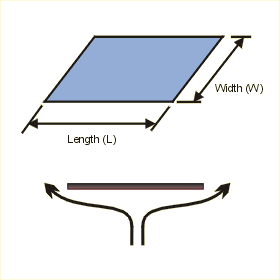Close

This page calculates the average heat transfer coefficient and plate temperature for an isothermal (constant temperature) heated horizontal flat plate's lower surface or a cooled plate's upper surface in a natural convection environment.The calculation is based on Nusselt number correlations.

The heat flow (q) from the plate is calculated as:

q = h A x (Tp - Ta)

Where h is the average heat transfer coefficient, A is the area of the plate, Tp is the plate temperature and Ta is the ambient fluid temperature. h is defined as:

h = Nu k / cl

Where Nu is the Nusselt Number, k the conductivity of the fluid and L the length of the plate. The Nusselt number is calculated as:

For Ra values between 105 and 1011
Nu = 0.27 (Gr* Pr)0.25

For the above equations Ra is the Rayleigh number, Gr is the Grashof number, and Pr is the Prandtl number and are calculated using fluid properties as follows:

Ra = (Gr * Pr)
Pr = kinematic viscosity / thermal diffusivity
Gr = gravity x (coefficient of expansion) x (Tp - Ta) x cl3 / (kinematic viscosity)2

Where cl is the characteristic dimension which is calculated using:

cl = area / perimeter

In addition, you must define the fluid properties at the film temperature Tf defined as follows, except for the density which is defined at the reference temperature of 20 C.

Tf = (Tp + Ta) / 2

The fluid density at the film temperature is automatically calculated from the following relationship based on the perfect gas law:

Rho = Rhoref (Tref +273) / (Tf + 273)

References

Hatfield, D. W. and D. K. Edwards, Edge and Aspect Ratio Effects on Natural Convection from the Horizontal Heated Plate Facing Downwards, Int. J. Heat Transfer, vol. 24, p. 1019, 1981.

Fujii, T. and H. Imura, Natural Convection Heat Transfer from a Plate with Arbitrary Inclination, Int. J. Heat Mass Transfer, vol. 15, p. 755, 1972.

Clifton, J. V. and A. J. Chapman, Natural Convection on a Finite-Size Horizontal Plate, Int. J. Heat Mass Transfer, vol. 12, p. 1573, 1969.

Holman, J.P., Heat Transfer, 7th ed., McGraw Hill Book Company, New York, 1990. p. 333 - 352